• 我看了答案还是有些不能完全理解，于是又去b站翻了翻教程基础DP，其中提到记忆化的递归（也称记忆化搜索），相当于结合了dp和递归的优点（这时我又觉得比DP还厉害），然后就准备写写记忆化递归。 目录 ​ 1.记忆化...

前言
​		前一篇博客写到入门的dp算法，后来又遇到一个奇怪的变种题目，同样也是可以用dp写的（至少标签是有动态规划）。我看了答案还是有些不能完全理解，于是又去b站翻了翻教程基础DP，其中提到记忆化的递归（也称记忆化搜索），相当于结合了dp和递归的优点（这时我又觉得比DP还厉害），然后就准备写写记忆化递归。

目录
​	1.记忆化递归的解释与分析
​	2.记忆化递归的应用

一、记忆化递归的解释与分析
前面说道它结合了dp和递归的优点，分别是记忆化和逻辑清晰易懂。
下面还是结合斐波那契数列的来理解：
F(0)=F(1)=1;
F(n)=F(n-1)+F(n-2) (n≥2,n∈N*);
这里直接给出函数代码，再进行解释：
int F(int n){
if(n<2)f[n]=1;			//这里f[]是储存数据的数组
else if(f[n]==0)		//这里是重点
f[n]=F(n-1)+F(n-2);
return f[n];
}

代码解释：
第3行中else if的条件很关键：当f[n]没被计算过，就计算一次。也就是说，如果f[n]已经被计算过储存起来了，那就直接用储存的数据，便不用再计算了。
分析优势：
相对于递归，逻辑清晰易懂，就不用说了。
主要是相对于dp的优势。从上一篇知道dp是将基础全部算出来，然后在这个基础上计算出我们要的那个值，减少了相对普通递归的重复计算。
记忆化递归则更加”投机取巧“了，它只计算了需要用的值并储存起来，而其它不会用到的值不去计算，最大化地减少了计算。打个比方，dp就相当于计算了一个方阵上所有的点（无论有没有利用价值），而记忆化递归相当于计算了方阵上有价值的点，因此记忆化递归的运行时间可能比dp还要短。（注意只是可能，因为斐波那契数列无论是dp还是记忆化递归，都是要把前面的值全部算出来的）

二、记忆化递归的应用
感觉没啥写的,就拿分配宝藏来写shui一写shui吧。题目在这里。
#include <stdio.h>
int W,sum,d;
int max(int a,int b);
int main(void){
int n;
scanf("%d",&n);
for (int i = 1; i <= n; ++i){
scanf("%d",&W[i]);
sum+=W[i];
}
printf("%d\n",sum-2*f(n,sum/2));
return 0;
}
if(ret==0){					//这里就是判断是否被计算过
else
}
return ret;
}
int max(int a,int b){
int m = a;
if( a < b) m = b;
return m;
}

我交上去的时候显示运行时间和用dp写的一样。


展开全文算法 c语言
• 下列图片主要解释从一个递归问题，可以用记忆化搜索来优化，也可用动态规划来解决。 拿斐波那契数列数列举例： 递归树如下，可以看到存在大量重复计算 如果设置一个全局的数组，初始化全为 -1，用来来保存子...

下列图片主要解释从一个递归问题，可以用记忆化搜索来优化，也可用动态规划来解决。拿斐波那契数列数列举例：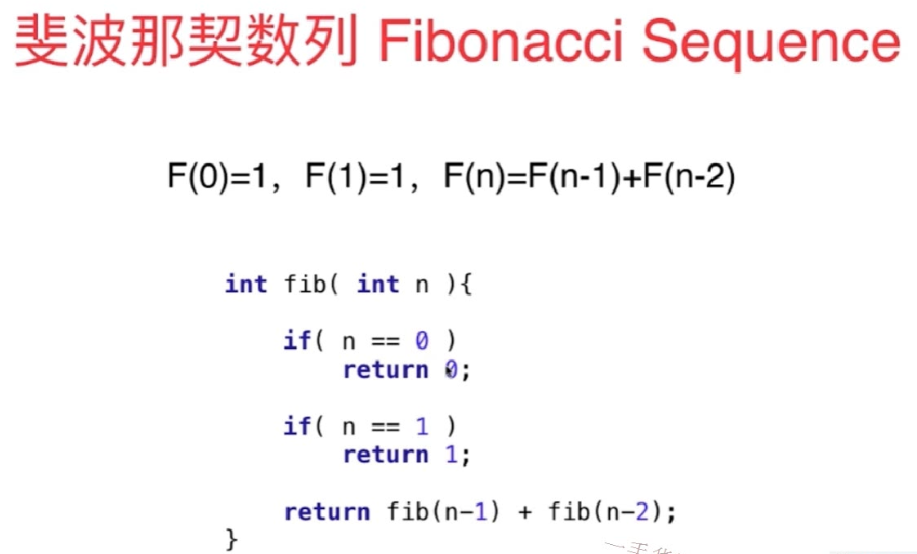递归树如下，可以看到存在大量重复计算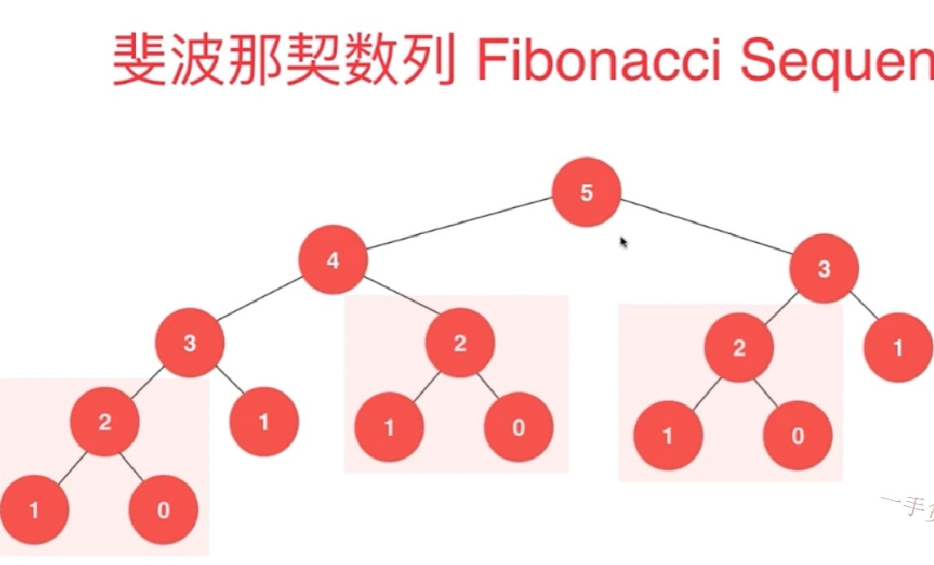如果设置一个全局的数组，初始化全为 -1，用来来保存子问题的答案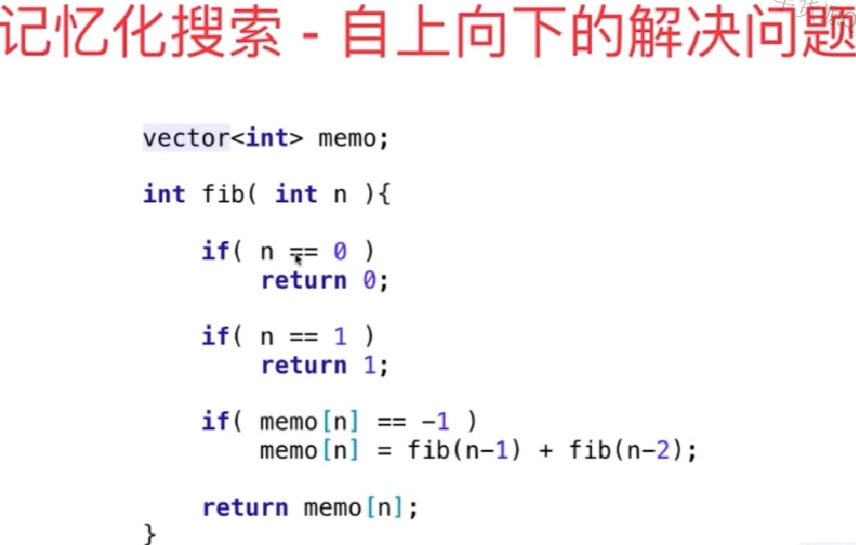记忆化搜索和递归大致思路一样，是一种自顶向下的思路，而动态规划则是一种自底向上的思路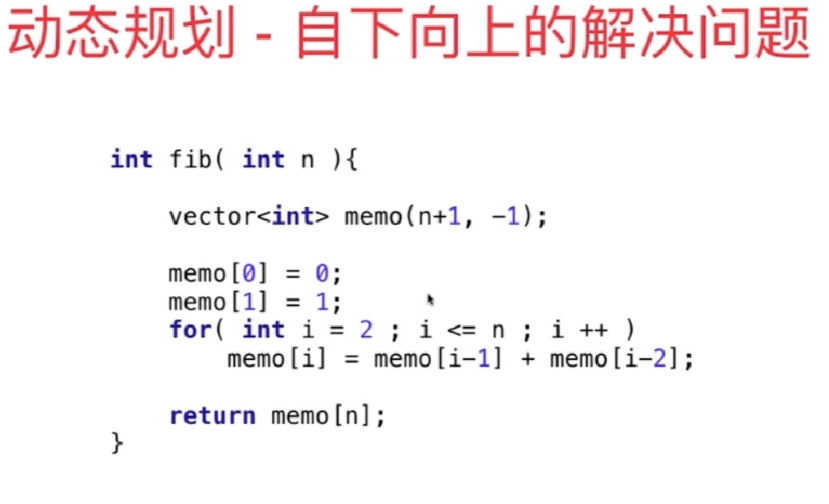那么究竟什么是动态规划呢？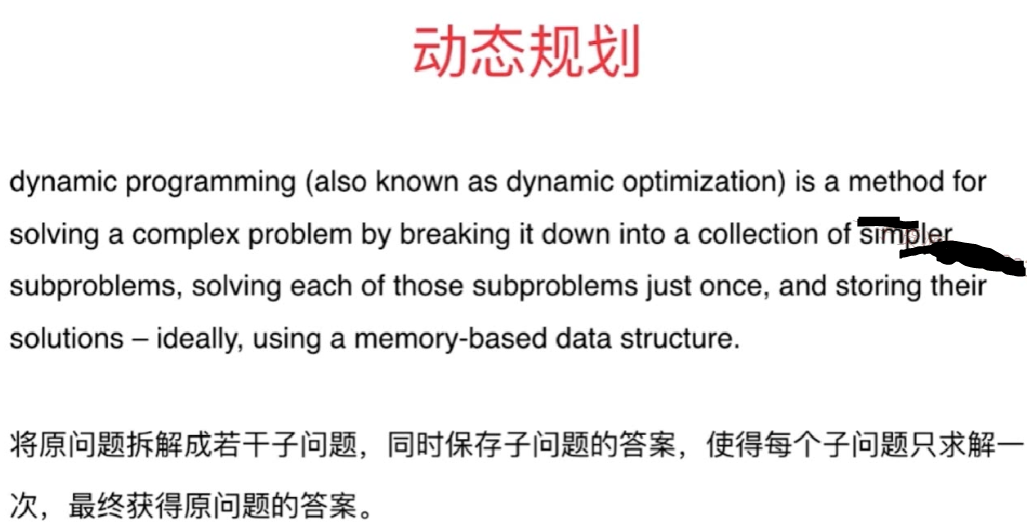三者之间的联系，一般考虑一个问题，我们习惯是自顶向下的思路，将大问题缩小规模，分成若干同类型小问题，这便是递归。如果我们反向思考，便是动态规划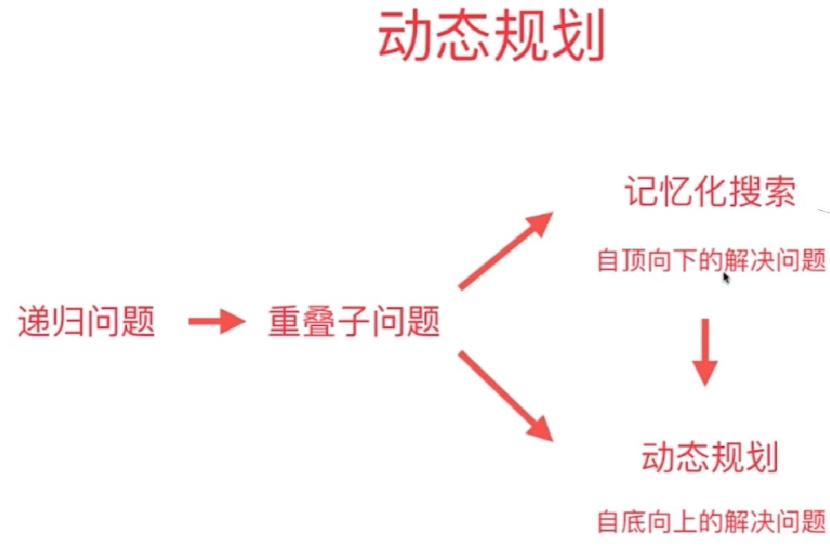展开全文动态规划
• 递归记忆化搜索 题目描述 对于一个递归函数w(a,b,c)w(a,b,c) 如果a≤0 or b≤0 or c≤0就返回值1. 如果a>20 or b>20 or c>20就返回w(20,20,20) 如果a<b并且b<c 就返回w(a,b,c-1)+w(a,b-1,c-1)-w(a,...
递归的记忆化搜索
题目描述
对于一个递归函数w(a,b,c)w(a,b,c)
如果a≤0 or b≤0 or c≤0就返回值1.
如果a>20 or b>20 or c>20就返回w(20,20,20)
如果a<b并且b<c 就返回w(a,b,c-1)+w(a,b-1,c-1)-w(a,b-1,c)
其它的情况就返回w(a-1,b,c)+w(a-1,b-1,c)+w(a-1,b,c-1)-w(a-1,b-1,c-1)
这是个简单的递归函数，但实现起来可能会有些问题。当a,b,ca,b,c均为15时，调用的次数将非常的多。你要想个办法才行.
对于w(30,-1,0)w(30,−1,0)既满足条件1又满足条件2，这种时候我们就按最上面的条件来。所以答案为1
输入格式会有若干行。并以-1,-1,-1结束。保证输入的数在[-9223372036854775808,9223372036854775807]之间，并且是整数。
输出若干行，每一行格式：
w(a, b, c) = ans

思路

对于一些递归问题， 总是会重复某些复杂的递归过程，可以通过引用一个数组来记录已经计算过的值从而避免重复递归。就叫做记忆化搜索。

代码
#include <iostream>
#include <cstdio>
#define INF 0x3f3f3f3f
using namespace std;
long long s;
long long w(int x,int y,int z){
if(x<=0||y<=0||z<=0) return 1;
else if(x>20||y>20||z>20) return w(20,20,20);
else if(s[x][y][z]!=INF) return s[x][y][z];
else if(x<y&&y<z){
s[x][y][z]=w(x,y,z-1)+w(x,y-1,z-1)-w(x,y-1,z);
return s[x][y][z];
}
else{
s[x][y][z]=w(x-1,y,z)+w(x-1,y-1,z)+w(x-1,y,z-1)-w(x-1,y-1,z-1);
return s[x][y][z];
}
int main(){
for(int i=0;i<25;i++)
for(int j=0;j<25;j++)
for(int q=0;q<25;q++)
s[i][j][q]=INF;
while(1){
long long a,b,c;
scanf("%lld%lld%lld",&a,&b,&c);
if(a==-1&&b==-1&&c==-1) break;
printf("w(%lld, %lld, %lld) = %lld\n",a,b,c,w(a,b,c));
}
return 0;
}



展开全文• 递归-记忆化搜索-动态规划 下面整理动态规划的相关问题，其动态规划和递归有着密切的联系，递归是自顶向下的过程，而动态规划是自底向上的过程。 所谓的顶指的是：复杂的大问题；所谓的底指的是：简单的子问题。 ...
递归-记忆化搜索-动态规划

下面整理动态规划的相关问题，其动态规划和递归有着密切的联系，递归是自顶向下的过程，而动态规划是自底向上的过程。 所谓的顶指的是：复杂的大问题；所谓的底指的是：简单的子问题。

LeetCode 70 ---爬楼梯

假设你正在爬楼梯。需要 n 步你才能到达楼顶。

每次你可以爬 1 或 2 个台阶。你有多少种不同的方法可以爬到楼顶呢？

注意：给定 n 是一个正整数。

示例 1：

输入： 2
输出： 2
解释： 有两种方法可以爬到楼顶。
1.  1 步 + 1 步
2.  2 步


示例 2：

输入： 3
输出： 3
解释： 有三种方法可以爬到楼顶。
1.  1 步 + 1 步 + 1 步
2.  1 步 + 2 步
3.  2 步 + 1 步


下面依次用三种方法进行实现，可以对比一下，会发现其不同点。

具体代码：

/*
//方法一：采用递归的方式---超时
class Solution {
public:
int climbStairs(int n) {
if(n==0)
return 1;
if(n==1)
return 1;
return climbStairs(n-1)+climbStairs(n-2);
}
};

//方法二：这是记忆化搜索的方法，能通过，但是还是自顶向下的方式
class Solution {
private:
vector<int>memo;
int climb(int n)
{
if(n==0)
return 1;
if(n==1)
return 1;
if(memo[n]==-1)
memo[n]=climb(n-1)+climb(n-2);
return memo[n];
}

public:
int climbStairs(int n) {
memo=vector<int>(n+1,-1);
return climb(n)；
}
};
*/

//方法三：这是动态规划的方法--实现了自底向下的方法（从简单问题向复杂问题进行）
class Solution {
public:
int climbStairs(int n) {
vector<int>memo(n+1,-1);
if(n==0||n==1)
return 1;

memo=1;
memo=1;

for(int i=2;i<=n;i++)
memo[i]=memo[i-1]+memo[i-2];

return memo[n];

}
};



LeetCode 343---整数拆分

给定一个正整数 n，将其拆分为至少两个正整数的和，并使这些整数的乘积最大化。 返回你可以获得的最大乘积。

例如，给定 n = 2，返回1（2 = 1 + 1）；给定 n = 10，返回36（10 = 3 + 3 + 4）。

注意：你可以假设 n 不小于2且不大于58。

下面进行了三种方法的对比，代码如下：

具体代码：

class Solution {
private:
//方法一：递归的实现----超时未通过
int BreakLine1(int n)
{
if(n==1)   //无法分割，返回1
return 1;

int res=0;
for(int i=1;i<=n-1;i++)
//分割成i和n-i两部分
res=max(max(res,i*BreakLine1(n-i)),i*(n-i));
return res;
}

//方法二：记忆化搜索的方法--自顶向下

vector<int>memo;
int BreakLine2(int n)
{
memo=1;

if(memo[n]==-1)
{
int res=-1;
for(int i=1;i<=n-1;i++)
//分割成i和n-i两部分
res=max(max(res,i*BreakLine2(n-i)),i*(n-i));
memo[n]=res;
}
return memo[n];
}

//方法三：动态规划方法--自底向上
int BreakLine3(int n)
{
memo=1;
for(int i=2;i<=n;i++)            //注意结束条件！！！能取到n
for(int j=1;j<=i-1;j++)
//分成了两部分j和i-j。
memo[i]=max(memo[i],max(j*memo[i-j],j*(i-j)));

return memo[n];
}
public:
int integerBreak(int n) {
memo=vector<int>(n+1,-1);
return BreakLine3(n);
}
};


LeetCode 198---打家劫舍

你是一个专业的小偷，计划偷窃沿街的房屋。每间房内都藏有一定的现金，影响你偷窃的唯一制约因素就是相邻的房屋装有相互连通的防盗系统，如果两间相邻的房屋在同一晚上被小偷闯入，系统会自动报警。

给定一个代表每个房屋存放金额的非负整数数组，计算你在不触动警报装置的情况下，能够偷窃到的最高金额。

示例 1:

输入: [1,2,3,1]
输出: 4
解释: 偷窃 1 号房屋 (金额 = 1) ，然后偷窃 3 号房屋 (金额 = 3)。
偷窃到的最高金额 = 1 + 3 = 4 。


示例 2:

输入: [2,7,9,3,1]
输出: 12
解释: 偷窃 1 号房屋 (金额 = 2), 偷窃 3 号房屋 (金额 = 9)，接着偷窃 5 号房屋 (金额 = 1)。
偷窃到的最高金额 = 2 + 9 + 1 = 12 。


下面用三种方法进行实现

代码如下：

class Solution {
private:
//方法一：递归实现---超时
int toudao1(vector<int>& nums,int index)
{
if(index>=nums.size())
return 0;
int res=0;
for(int i=index;i<nums.size();i++)
res=max(res,nums[i]+toudao1(nums,i+2));
return res;
}

//方法二：记忆化搜索
vector<int>memo;
int toudao2(vector<int>nums,int index)
{
if(index>=nums.size())
return 0;
if(memo[index]!=-1)
return memo[index];
int res=0;
for(int i=index;i<nums.size();i++)
res=max(res,nums[i]+toudao2(nums,i+2));
memo[index]=res;
return res;
}

//方法三：动态规划--自底向上求解  ---状态定义：index表示偷盗[index,,,,,nums.size()]的范围，如果状态定义
//换了下面的程序是需要重新改写的。（时刻注意问题的规模---从小规模问题的解去求取大规模问题的解）
int toudao3(vector<int>nums,int index)
{
int n=nums.size();
memo[n-1]=nums[n-1];

for(int i=n-2;i>=0;i--)
//求解memo[i]
for(int j=i;j<n;j++)
memo[i]=max(memo[i],nums[j]+(j+2<n?memo[j+2]:0));  //这个条件需要注意，j+2有可能超出范围需要注意一下！！！

return memo;

}

public:
//这个偷盗的问题需要注意偷盗顺序。
int rob(vector<int>& nums) {
if(nums.size()==0)
return 0;

memo=vector<int>(nums.size(),-1);

}
};
展开全文数据结构与算法 动态规划
• 边界条件与递归方程是递归函数的两个要素。 1）阶乘函数 直接打板子： Int fac(int n) { If (n==0) return 1; Else return n*fac(n-1); } 这里，第一句的if是边界条件，第二句是递归方程。0的阶乘为1，n的...
• ## 递归+记忆化搜索

千次阅读 2017-10-29 07:22:35
边界条件与递归方程是递归函数的两个要素。 1）阶乘函数 直接打板子： Int fac(int n) { If (n==0) return 1; Else return n*fac(n-1); } 这里，第一句的if是边界条件，第二句是递归方程。0的阶乘为1，n的阶乘为（n-...
• 对于一个递归函数w(a,b,c)w(a,b,c) 如果a≤0or b≤0or c≤0就返回值1. 如果a>20or b>20or c>20就返回w(20,20,20) 如果a<b并且b<c就返回w(a,b,c-1)+w(a,b-1,c-1)-w(a,b-1,c) 其它的情况就返回w(a-...
• 最好的方法就是用记忆化搜索，用数组将值记录下来，当搜到已经计算过的值时直接使用就行了，避免再一次递归计算，这样会节省很多时间。 代码如下：http://blog.csdn.net/non_cease/article/details/6817914 #include...c++ c
• 这就是所谓的记忆化搜索。 #include #include #include #include #define mm(a,n) memset(a,n,sizeof(a)) #define ll long long #define maxn 100 using namespace std; int a[maxn][maxn],N,total,dp[maxn]...
• 今天做洛谷P1434 [SHOI2002]滑雪 的时候仔细想了想记忆化搜索 现在总结一下 为了描述问题的某一状态，必须用到该状态的上一状态，而描述上一状态，又必须用到上一状态的上一状态……这种用自已来定义自己的方法，...
• 递归函数运行时分为函数 前进段 和 返回段 ，真正明白并时刻记住这个才真正掌握了递归。...记忆化搜索：解决了递归时大量的重复计算（通过将每次计算值记录入数组）本质：空间换时间。递推：推出公式。（感觉
• 搜索分析（DFS、BFS、递归记忆化搜索） 1、线性查找 在数组a[]={0,1,2,3,4,5,6,7,8,9,10}中查找1这个元素。 （1）普通搜索方法，一个循环从0到10搜索，这里略。 （2）递归（从中间向两边） 1 //递归...
• 题意已经很清楚了，直接搜索就行了，不过需要记忆化，不然会超时，就是用一个vis数组保存上次搜索过的路，这样一来，曾经搜索过的路，就不需要再走了。。。 AC代码： #include #include #include #include ...
• 任何一个动态规划都是某一种暴力递归的优化求解，故先从暴力递归开始做，改成记忆化搜索（傻缓存），再到动态规划 提示：以下是本篇文章正文内容，下面案例可供参考 一、例子 给一个数组，例如arr[]={2,3,5,10},2,3...java
• #include #include #include   int n, m;  int Map;  int dp;  int deta = {{1, 0}, {-1, 0}, {0, 1},{0, -1}}; int max(int x,int y) {  returnx>=y?...int solve(intdfs c语言
• + 记忆化搜索，本题也可以采用动态规划来解，下面贴递归 + 记忆化搜索的代码: #include #include #include #include using namespace std; const int maxn = 105; int map[maxn][maxn]; int dir ...
• 点击打开链接 函数 Description 计算ackerman函数值: Input 第一行为两个数，即M和N，其中0 Output 输出ack(m,n)的值。 Sample Input ...考虑记忆化搜索进行优化，利用数组对值进行储存，后面的值直
• 记忆化搜索3.记忆化搜索转化为动态规划 爬楼梯问题 题目描述：假设你正在爬楼梯。需要 n 阶你才能到达楼顶。 每次你可以爬 1 或 2 个台阶。你有多少种不同的方法可以爬到楼顶呢？ 例子： 输入： 2 输出： 2 解释：...前端 算法 动态规划
• 递归递归调用从哪里开始的，执行最后一定要返回到这个调用的地方。 举例更好理解：（二叉树递归求所有节点的个数） 模型： F(b)=0 若b=NULL F(b)=F(b-&amp;amp;gt;lchild)+F(b-&amp;amp;gt;rchild)+1...算法 动态规划
• 0. 概念 将原问题拆解成若干子问题，同时保存子问题的答案，...在递归和带记忆的递归（原理和例子）这篇博文中我们讨论了计算斐波那契数列的普通递归方法和加了记忆的递归方法(即记忆化搜索)，这里接着讨论如何使用动...